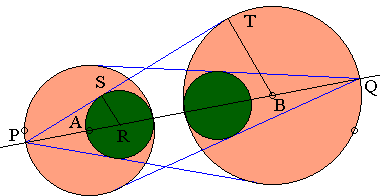# The Squinting Eyes Theorem: What Is It? A Mathematical Droodle

ExplanationThe applet suggests a statement reminiscent of the Eyeball theorem. To distinguish between the two, I'll call the current one

### The Squinting Eyes Theorem

Let there be two circles C(A, RA) and C(B, RB), one with center A and radius RA, the other with center B and radius RB. Let P and Q be the farthest points of the two circles, as on the diagram below. Draw the tangents from P to C(B, RB) and from Q to C(A, RA). Whenever the construction is possible, it leads to two "isosceles triangles" with one side a circular arc. The fact is that the "incircles" of the two triangles are always equal, i.e., have the same radius.### Proof

Let PT be tangent to C(B, RB), so that PT is perpendicular to BT. Let C(R, RS) be one of the two circles in question, and assume RS is also perpendicular to PT. From the similarity of triangles PTB and PSR,

PB/BT = PR/RS,

from where

(RA + AB)/RB = (2·RA - RS)/RS.

Solving this for RS gives

RS = 2·RA·RB/(RA + RB + AB).

In the same manner we could find the radius of the second "incircle". However, there is no need to. The formula for RS is symmetric in A and B, which means that the result would not change if we swapped A and B. Because of the symmetry, we can claim that the radius of the second circle is defined by exactly same formula, i.e. the two radii are indeed equal.

This Sangaku problem has been written on a tablet in 1842 in the Aichi prefecture [Temple Geometry, p. 82].

### References

1. H. Fukagawa, D. Pedoe, Japanese Temple Geometry Problems, The Charles Babbage Research Center, Winnipeg, 1989

Write to:

Charles Babbage Research Center
P.O. Box 272, St. Norbert Postal Station
Winnipeg, MB

2. H. Fukagawa, A. Rothman, Sacred Geometry: Japanese Temple Geometry, Princeton University Press, 2008, p. 102
3. P. Yiu, Geometric Art Design## Sangaku

### Problems with Ophthalmological Connotations

• The Eyeball Theorem
• Eye-to-Eye Theorem I
• Eye-to-Eye Theorem II
• Eyeballing a ball
• Praying Eyes Theorem
• Focus on the Eyeball Theorem
• Eyeball Theorem Rectified
• Bespectacled Eyeballs Extension
• Shedding Light on the Ball for Eyeballing
• Eyeballs Projected
• Archimedean Siblings out of Wedlock, i.e., Arbelos
• Rectified, Halved, Sheared, Eyeballs Still Surprise
•